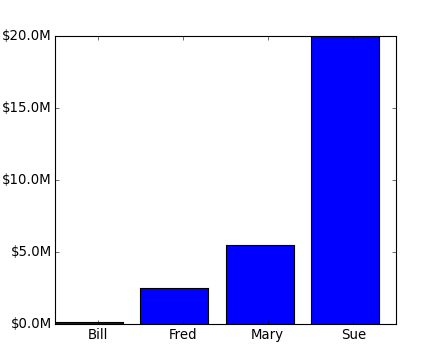#### Previous topic

pylab_examples example code: custom_figure_class.py

#### Next topic

pylab_examples example code: customize_rc.py

# pylab_examples example code: custom_ticker1.py¶```#!/usr/bin/env python

"""
The new ticker code was designed to explicitly support user customized
ticking.  The documentation
http://matplotlib.org/matplotlib.ticker.html details this
process.  That code defines a lot of preset tickers but was primarily
designed to be user extensible.

In this example a user defined function is used to format the ticks in
millions of dollars on the y axis
"""
from matplotlib.ticker import FuncFormatter
import matplotlib.pyplot as plt
import numpy as np

x =     np.arange(4)
money = [1.5e5, 2.5e6, 5.5e6, 2.0e7]

def millions(x, pos):
'The two args are the value and tick position'
return '\$%1.1fM' % (x*1e-6)

formatter = FuncFormatter(millions)

fig, ax = plt.subplots()
ax.yaxis.set_major_formatter(formatter)
plt.bar(x, money)
plt.xticks( x + 0.5,  ('Bill', 'Fred', 'Mary', 'Sue') )
plt.show()
```

Keywords: python, matplotlib, pylab, example, codex (see Search examples)